# Unity移动端软阴影技术总结

## RenderTexture 方法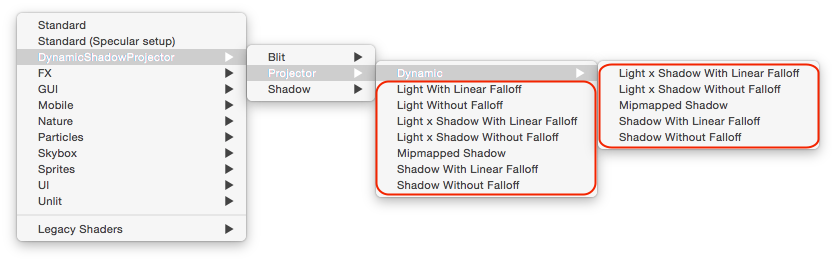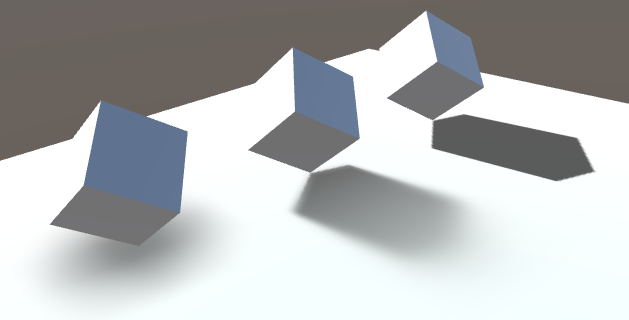MipMap具体实现方式可以参考：Percentage Closer Filter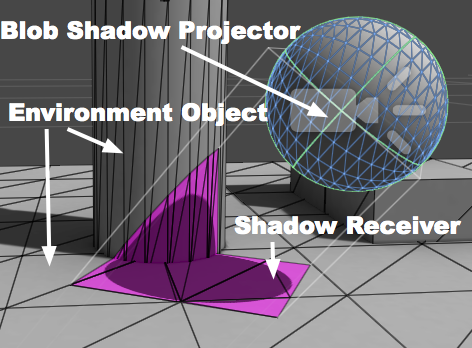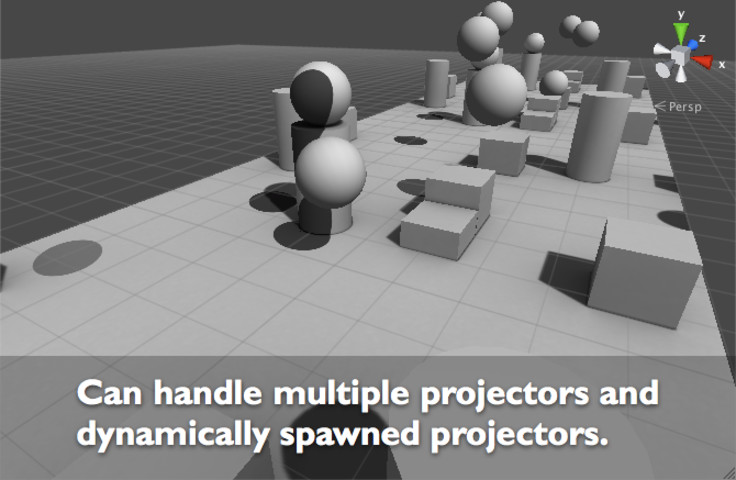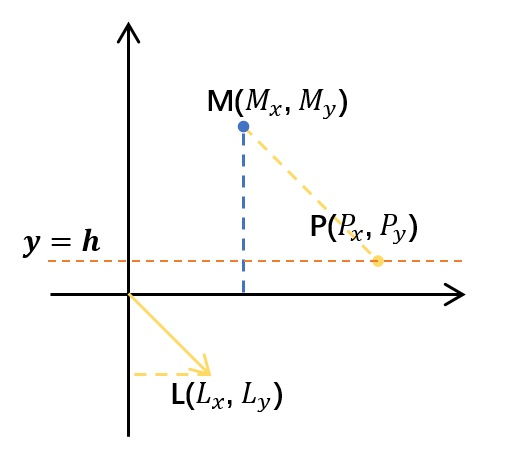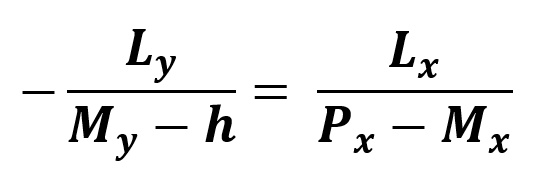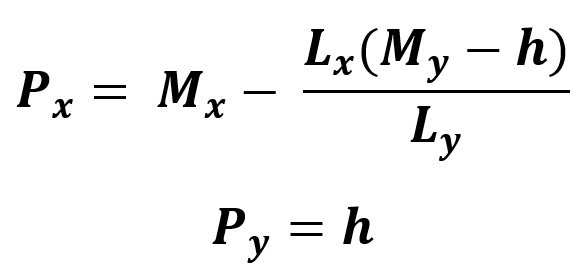Shader "PlanarShadow/Shadow"
{
Properties
{
_MainTex ("Texture", 2D) = "white" {}

}

{
Tags{ "RenderType" = "Opaque" "Queue" = "Geometry+10" }
LOD 100

Pass
{
CGPROGRAM

#pragma vertex vert
#pragma fragment frag
// make fog work
#pragma multi_compile_fog

#include "UnityCG.cginc"

struct appdata
{
float4 vertex : POSITION;
float2 uv : TEXCOORD0;
};

struct v2f
{
float2 uv : TEXCOORD0;
UNITY_FOG_COORDS(1)
float4 vertex : SV_POSITION;
};

sampler2D _MainTex;
float4 _MainTex_ST;

v2f vert (appdata v)
{
v2f o;
o.vertex = UnityObjectToClipPos(v.vertex);
o.uv = TRANSFORM_TEX(v.uv, _MainTex);
UNITY_TRANSFER_FOG(o,o.vertex);
return o;
}

fixed4 frag (v2f i) : SV_Target
{
// sample the texture
fixed4 col = tex2D(_MainTex, i.uv);
// apply fog
UNITY_APPLY_FOG(i.fogCoord, col);
return col;
}

ENDCG
}

Pass
{
Blend SrcAlpha  OneMinusSrcAlpha
ZWrite Off
Cull Back

Stencil
{
Ref 0
Comp Equal
//Pass IncrSat
Pass Invert
Fail Keep
ZFail Keep
}

CGPROGRAM

#pragma vertex vert
#pragma fragment frag

float4 _WorldPos;

struct appdata
{
float4 vertex : POSITION;
};

struct v2f
{
float4 vertex : SV_POSITION;
float3 xlv_TEXCOORD0 : TEXCOORD0;
float3 xlv_TEXCOORD1 : TEXCOORD1;
};

v2f vert(appdata v)
{
v2f o;

float3 worldpos = mul(unity_ObjectToWorld, v.vertex).xyz;
// _ShadowPlane.w = p0 * n  // 平面的w分量就是p0 * n
worldpos = worldpos + distance * lightdir.xyz;
o.vertex = mul(unity_MatrixVP, float4(worldpos, 1.0));
o.xlv_TEXCOORD0 = _WorldPos.xyz;
o.xlv_TEXCOORD1 = worldpos;

return o;
}

float4 frag(v2f i) : SV_Target
{
float3 posToPlane_2 = (i.xlv_TEXCOORD0 - i.xlv_TEXCOORD1);
float4 color;
color.xyz = float3(0.0, 0.0, 0.0);

// 王者荣耀的衰减公式

// 另外的阴影衰减公式
//color.w = 1.0 - saturate(distance(i.xlv_TEXCOORD0, i.xlv_TEXCOORD1) * _ShadowFalloff);

return color;
}

ENDCG
}
}
}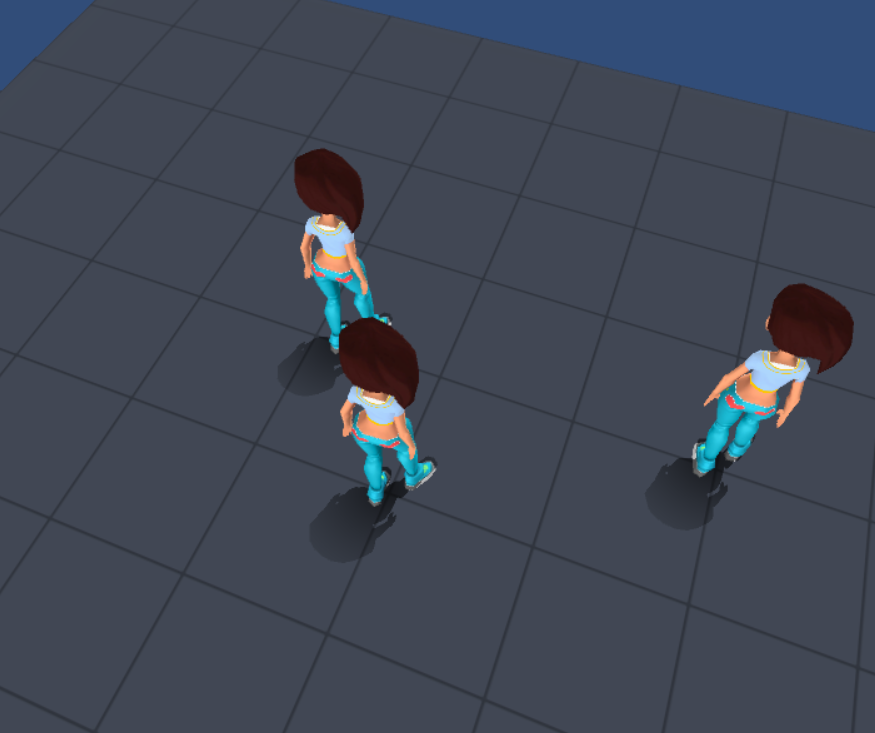## SDF渲染

SDF全称 Signed Distance Field Shadow ，该渲染是基于RayMarching实现的，也是一种新的实现方式，可以看到下图中物体的阴影随着距离由近到远也逐渐由清晰渐渐过渡到模糊的效果，表现更加自然而真实。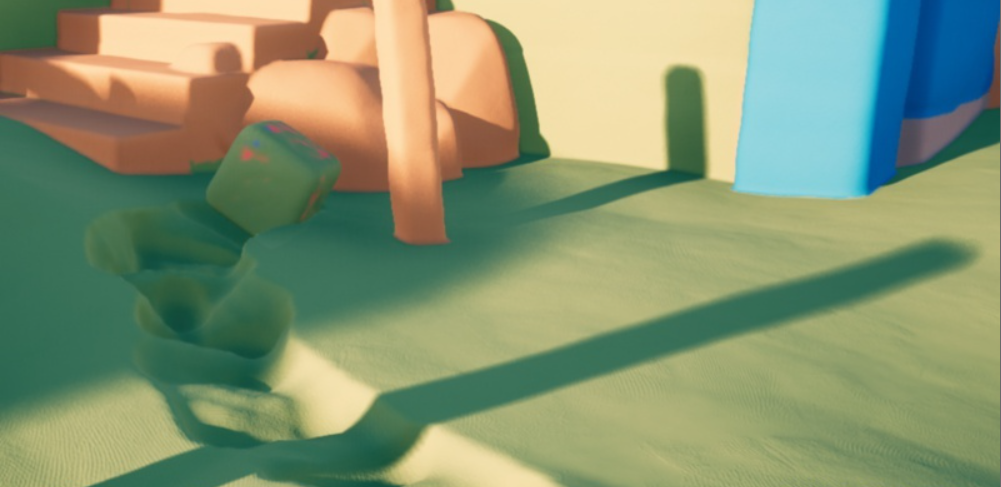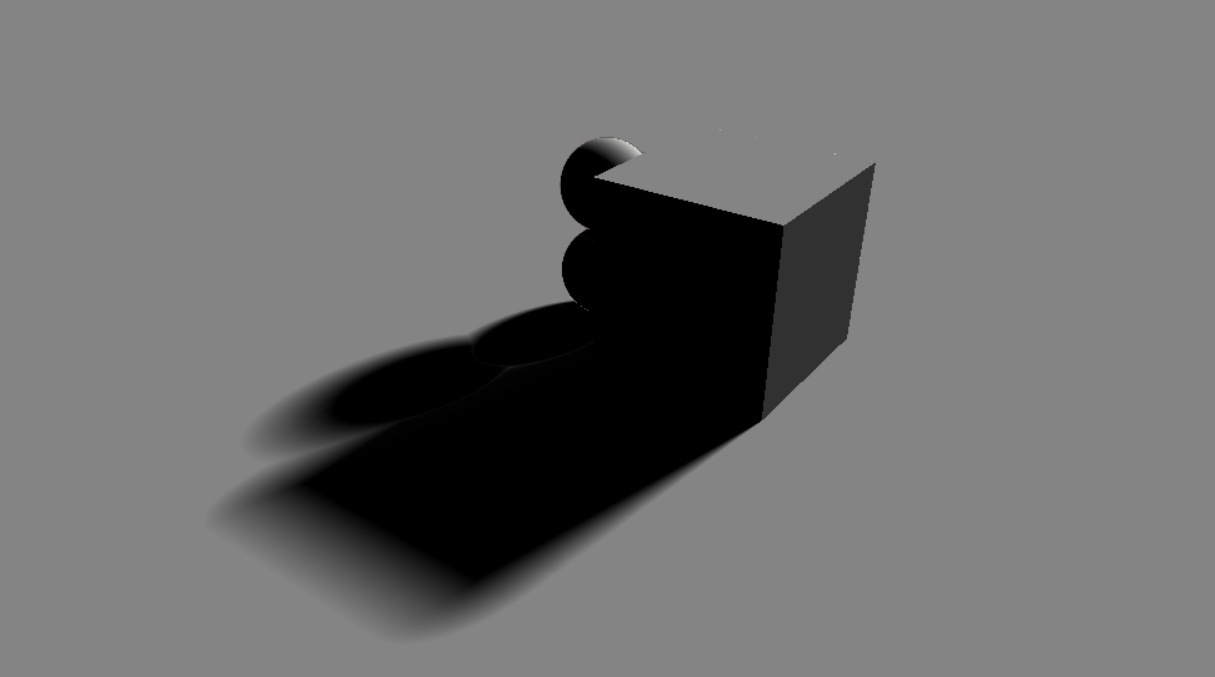float calcSoftshadow( float3 ro, float3 rd, in float mint, in float tmax)
{

float res = 1.0;
float t = mint;
float ph = 1e10;

for( int i=0; i<32; i++ )
{
float h = map( ro + rd*t );
float y = h*h/(2.0*ph);
float d = sqrt(h*h-y*y);
res = min( res, 10.0*d/max(0.0,t-y) );
ph = h;

t += h;

if( res<0.0001 || t>tmax )
break;

}
return clamp( res, 0.0, 1.0 );
}

float3 calcNorm(float3 p);

fixed4 raymarching(float3 rayOrigin, float3 rayDirection)
{

fixed4 ret = fixed4(0, 0, 0, 0);

int maxStep = 64;

float rayDistance = 0;

for(int i = 0; i < maxStep; i++)
{
float3 p = rayOrigin + rayDirection * rayDistance;
float surfaceDistance = map(p);
if(surfaceDistance < 0.001)
{
ret = fixed4(1, 0, 0, 1);
//增加光照效果

float3 norm = calcNorm(p);
ret = clamp(dot(-_LightDir, norm), 0, 1) * calcSoftshadow(p, -_LightDir,    0.01, 300.0);
ret.a = 1;

//
break;
}

rayDistance += surfaceDistance;
}
return ret;
}

//计算光照
//计算法线
float3 calcNorm(float3 p)
{
float eps = 0.001;

float3 norm = float3(
map(p + float3(eps, 0, 0)) - map(p - float3(eps, 0, 0)),
map(p + float3(0, eps, 0)) - map(p - float3(0, eps, 0)),
map(p + float3(0, 0, eps)) - map(p - float3(0, 0, eps))
);

return normalize(norm);
}


## 总结：

https://zhuanlan.zhihu.com/p/42781261

https://pan.baidu.com/s/1B4MlzK7VCnCLTNMzFtYNJg 密码：m1vl

01-08
10-02125105-13
12-273604
07-12763
08-256943
07-3198
10-07187
07-16687